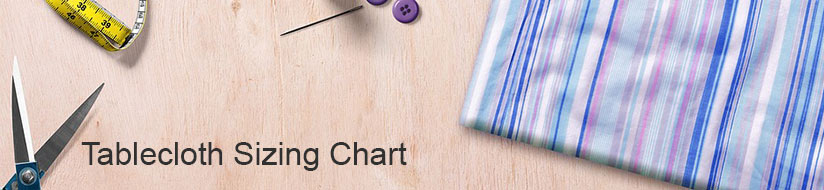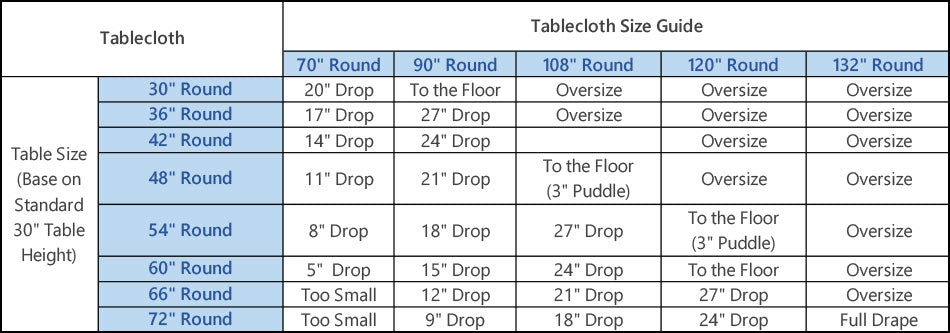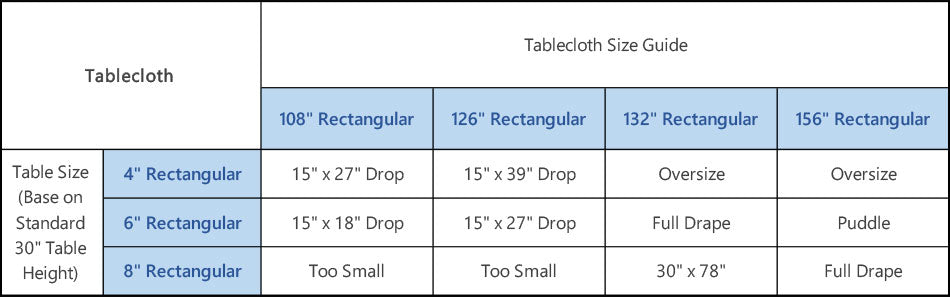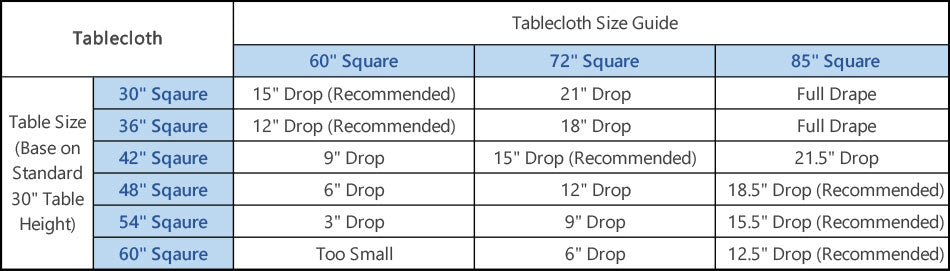Tablecloth Sizing ChartWe created the table linen sizing charts below as a helpful tool when you are determining the perfect tablecloth size for your round or rectangular tables.

It is easy to make purchasing decisions and implement design ideas if you know how to measure tablecloths that will match the style that you are looking for.

First:

Use a measure Tape to take an exact measurement. This will give you the size of the tabletop and the measurements you'll use to determine the tablecloth size you need.

There Are 3 Kinds of Tablecloths are available

• Rectangle and oval tables come in two standard widths. The most common is 36" and the other is 40" wide.
• For a round table you will to know the diameter. Measure the center of the table from one end to the other.
• Square tables range from 30" to 72". You can determine the size of your table by measuring one side.

Second

Choose the length of the Drop, which is the amount of material that will hang over the edge of the table, you want for your tablecloth. If you are Not Sure, then sit down at the table and with a ruler or tape measure find 8", measuring from the edge of the table. If this length isn't as long as you'd like, then try 10" and finally 12".Now that you know the size of your table and the drop length you want, you're ready to find the size of the tablecloth you'll need with the chart.

Understanding Tablecloth Drop Length Options

The material of a standard tablecloth needs to fall over the edge of the table. That material is called a drop and it impacts the length you need so the tablecloth fits your table and provides the look you desire.

Quick Reference for Finding Tablecloth Sizes

Using the chart below, the quick way to find the correct size for any standard size tablecloth. With the various options for drop length, the chart becomes a highly valuable tool for making the right purchase for your next tablecloth.

ROUND TABLECLOTH SIZING CHARTFor example, if your table is round and 120" in diameter and you want an 8" drop, you will first find your table size underneath the Table Shape and Size column. Next, move across the chart underneath the Tablecloth Size Drop 8" column. The tablecloth size you need is 136" in diameter.

Rectangle TABLECLOTH SIZING CHARTTo find the tablecloth size needed for a rectangle or oval table, you will use the length and width measurements you took earlier. In the chart listings you will see that the (40") widths are shown in parentheses in the Shape and Size column as well as each of the Tablecloth Size Drop columns.

If your table is a rectangle shape and measures 72" x 36" and you wish for a 10" drop, then the tablecloth size you need is 92" x 56". If your table has a 40" width instead of 36", then you would use the numbers in parentheses - (60") for a tablecloth size of 92" x 60".

Square TABLECLOTH SIZING CHARTTo find the tablecloth size needed for a 42" square table with a long drop, find the table shape and size underneath the Table Shape and Size column. Next, move across the chart underneath the Tablecloth Size Drop 12" column. The tablecloth size you need is a 58" x 58" square.

Oval TABLECLOTH SIZING CHART

For an oval table, you will use the rectangle size measurements and the correlating tablecloth sizes. Just be sure that when you purchase the tablecloth it is meant for an oval table and not a rectangle table.